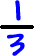Before, I showed you how to convert fractions to decimals...  But, only for certain, special kinds of fractions!  These fractions had denominators that were only made up of 2's and 5's.

LikeTo do these, you turned those denominators into powers of 10 to convert them to decimals.

Then, I told you that you needed another method to convert something likeSo, here is that method!  (Aren't you excited?)

To convert these critters into decimals, we use long division to divide the numerator by the denominator:

 Let's convertto a decimal.

Set it up and you'll see that the 3 doesn't divide in right away...  So, we need to use our knowledge of decimal division!Hey!  We're stuck in a repeating loop, aren't we?

Yep, these things always convert into repeating decimals!